Next: Transportation of the VHE Up: ph_rad Previous: Inverse Compton Scattering

# Pair Creation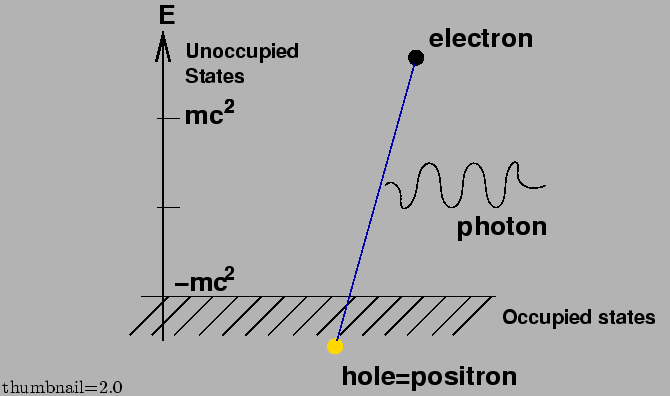Pair creation is a process of two photon collision to generate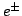pair,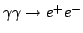. This process can be understood in the hole theory proposed by Paul Dirac in 1930 where the vacuum state is one with all negative energy electrons (corresponding to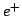) levels filled and all positive energy levels empty. It is possible for a negative-energy electron to absorb photons and be excited into a positive-energy state, as shown schematically in FIG. 5. If this occurs, we observe an electron of charge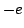and energy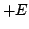and in addition a hole in the negative-energy sea. The hole registers the absence of an electron of chargeand energy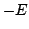and would be interpreted by an observer relative to the vacuum as the PRESENCE of a particle of charge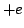and energy; that is the positron.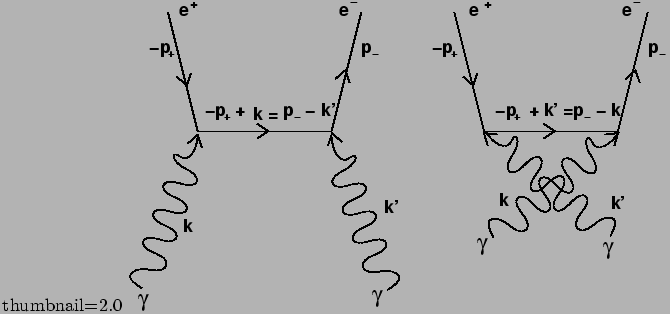This process is also described in the Feynman theory of positron where positrons are interpreted as a negative energy electron running backward in space-time. This interpretation leads to the famous Feynman diagram as shown in FIG. 6 by which the relevant cross section can be calculated in relatively straightforward manner.

The threshold energy of photons for pair creation is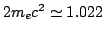MeV, as easily imagined by FIG. 5. Equivalently, the threshold CM energy squared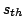defined by Eq. 26 is also given by the ones considered at recoiling electron rest system as. The pair creation process dominates the Compton scattering above this threshold energy.

The differential cross section can be obtained in CMS in relatively simpler manner. Under the UHE regime we are interested in here when we can neglect electron mass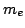in its second order, it is approximately given by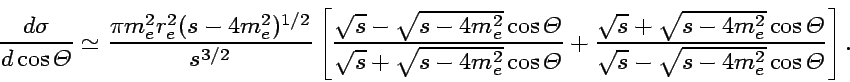(32)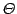is an emission angle in CMS.

SubsectionsNext: Transportation of the VHE Up: ph_rad Previous: Inverse Compton Scattering
Shigeru Yoshida
2002-07-18